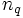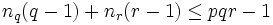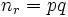# Order is product of three distinct primes implies normal Sylow subgroup

## Statement

Suppose$p < q < r$ are three distinct prime numbers and$G$ is a group of order$pqr$. Then, either the$q$-Sylow subgroup or the$r$-Sylow subgroup of$G$ is normal in$G$. In particular,$G$ has a normal Sylow subgroup.

## Proof

Given: Three distinct primes$p < q < r$. A group$G$ of order$pqr$.

To prove: Either$n_q = 1$ or$n_r = 1$.

Proof: Let$n_q, n_r$ denote the Sylow numbers for the primes$q,r$; in other words,$n_q$ is the number of$q$-Sylow subgroups of$G$ and$n_r$ is the number of$r$-Sylow subgroups of$G$.

1. By fact (1), the number of elements of order$q$ is$n_q(q-1)$ and the number of elements of order$r$ is$n_r(r-1)$. Since both these sets of elements are disjoint and they are all non-identity elements, we get$n_q(q-1) + n_r(r-1) \le pqr - 1$.
2.$n_r = 1$ or$n_r = pq$: By fact (2),$n_r|pq$. Thus,$n_r = 1, p, q, pq$. By fact (3),$n_r \equiv 1 \mod r$. Thus, if$n_r \ne 1$,$n_r > r$, so$n_r = pq$.
3.$n_q = 1$ or$n_q \ge r$: By fact (2),$n_q|pr$. Thus,$n_q = 1,p,r,pr$. By fact (3),$n_q \equiv 1 \mod q$. Thus, if$n_q \ne 1$,$n_q > q$. Thus$n_q = r, pr$. In either event,$n_q \ge r$.
4. If$n_q \ne 1$ and$n_r \ne 1$, we have a contradiction: If$n_q \ne 1$,$n_q \ge r$, and if$n_r \ne 1$,$n_r = pq$. Thus,$n_q(q-1) + n_r (r-1) \ge r(q - 1) + pq(r- 1) = pqr + r(q-1) - pq$. Also, note that$r(q - 1) = p(q - 1) + (r-p)(q-1) \ge p(q-1) + 2(q-1) > p(q-1) + p = pq$. Thus,$n_q(q-1) + n_r(r-1) > pqr$, a contradiction to the conclusion of step (1).